# 树莓派DIY自带特效的智能安全门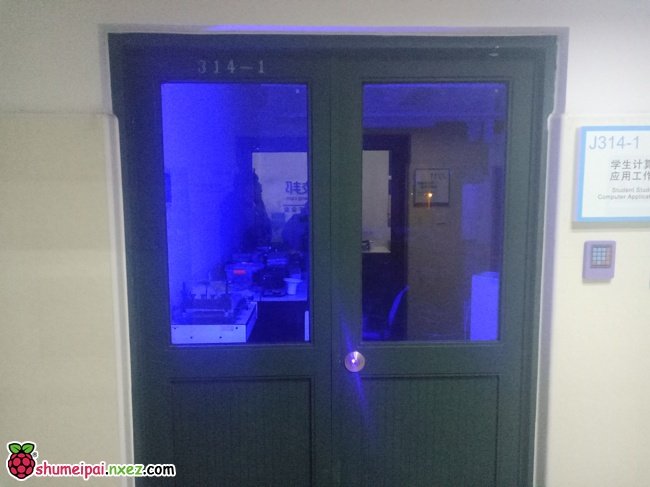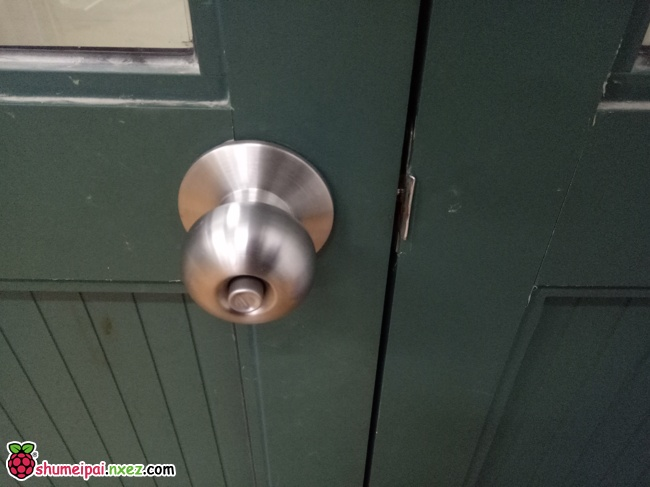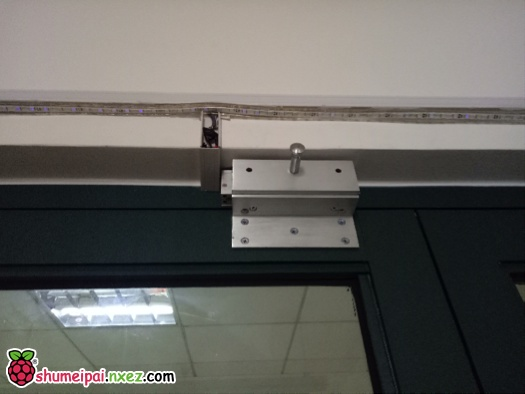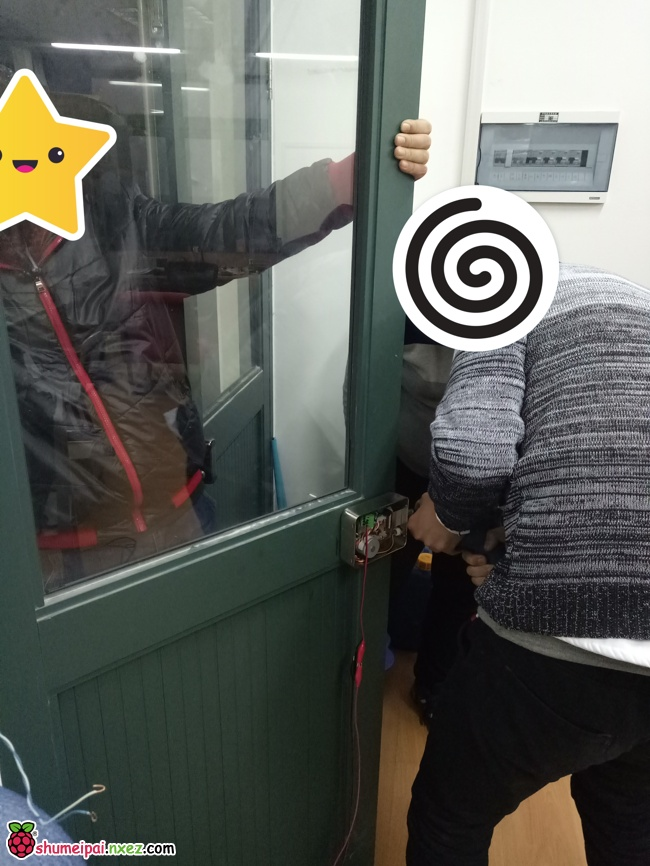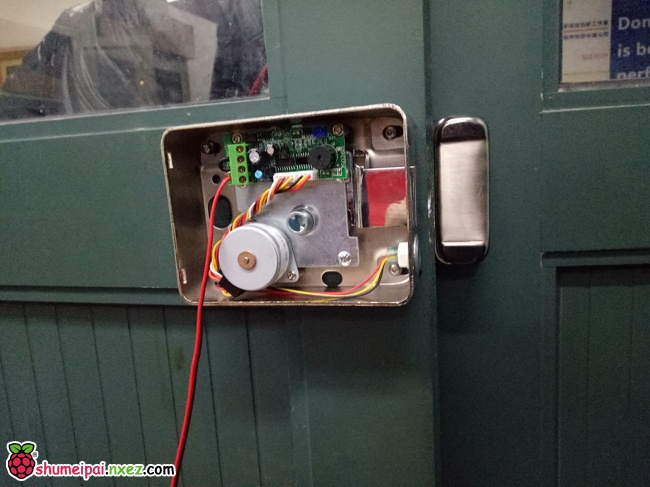（做的时候其实是先调试好了锁才考虑用什么方式走线的……一开始打算在门上钻孔走内部，但是不好弄，就直接拉网线走门边控制）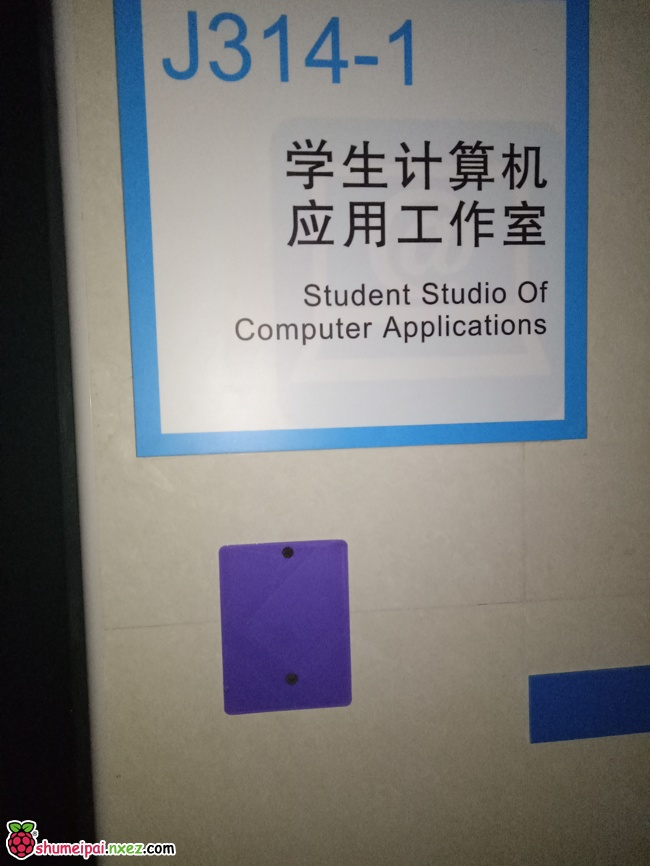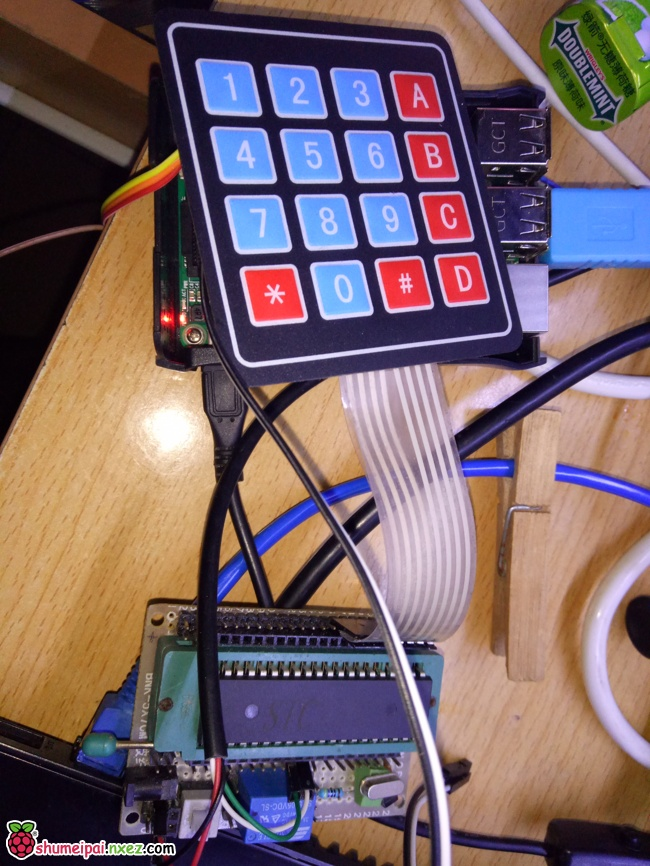```#include "STC15W404AS.h"
#include <intrins.h>

#define key_port P1	//键盘接口定义
sbit key_port_0=key_port^0;
sbit key_port_1=key_port^1;
sbit key_port_2=key_port^2;
sbit key_port_3=key_port^3;
sbit BEEP=P5^5;		//蜂鸣器

sbit OPEN=P5^4;		//开门按键
/*******************************
STC15W404单片机一毫秒延时函数
*******************************/
void delay_ms(unsigned int ms)
{
unsigned char i, j;
while(--ms){
_nop_();
_nop_();
_nop_();
i = 11;
j = 190;
do
{
while (--j);
} while (--i);
}
}
/**************************
串口发送一个字符
**************************/
void com_send_dat( unsigned char dat)
{
SBUF=dat;
while (TI== 0);
TI= 0 ;
}

void init_com(void)		//T2_9600bps@11.0592MHz
{
SCON = 0x50;
AUXR |= 0x01;
AUXR |= 0x04;
T2L = 0xE0;
T2H = 0xFE;
AUXR |= 0x10;
}
/**************************
键盘扫描函数
**************************/
unsigned char keyscan(void)
{
unsigned char key,i;
unsigned char code key_table={0xeb,0x77,0x7b,0x7d,0xb7,0xbb,0xbd,0xd7,0xdb,0xdd,0x7e,0xbe,0xde,0xee,0xe7,0xed};

key=0xFF;							//输出初始化值
key_port=0x0f;						//确定行列位置
if(key_port==0x0f) return(255);		//无键按下返回0
delay_ms(5);              			//调用延时函数 ，目的是去前沿键抖。
if(key_port==0x0f) return(255);		//再次判断。目的是确保检测正确
else
{
for(i=0;i<4;i++)	//以下为经典的计算键值（判断闭合键所在的位置）
{
key_port=_cror_(0x7f,i);
if(key_port_0==0) break;
if(key_port_1==0) break;
if(key_port_2==0) break;
if(key_port_3==0) break;
}
key=key_port;		//取得键值
for(;key_port!=0x0f;key_port=0x0f);			//等待键松开，目的是去后沿键抖
for(i=0;key_table[i]!=key && i<16;i++);		//查表取key的值0-F
key=i;
return(key);				//带键值返回主调函数
}
}

/**************************
键盘扫描测试主函数
**************************/
void main(void)
{
unsigned char key,nok;
init_com();			//串口初始化
nok=0;
while(1)
{
key=keyscan();
if(key!=0xff)
{
BEEP=0;
com_send_dat(key+0x30);
delay_ms(20);
BEEP=1;
}

if(OPEN==0)		//如果从里面按下了开关则发送一个字符串
{
com_send_dat('Y');
com_send_dat('E');
com_send_dat('S');
com_send_dat('O');
com_send_dat('P');
com_send_dat('E');
com_send_dat('N');
while(!OPEN);
delay_ms(10);
}

}
}
```

```#include <STC12C5A60S2.h>

#include <intrins.h>

sbit IN1=P1^0;		//霍尔传感器1
sbit IN2=P1^1;		//霍尔传感器2
sbit OUT=P2^0;		//门控制

sbit RELAY=P2^1;	//继电器
bit OFLAG = 0;		//状态标记

void Delay100ms(unsigned int t)		//@11.0592MHz
{
unsigned char i, j, k;

while(--t)
{
//_nop_();
//_nop_();
i = 5;
j = 52;
k = 195;
do
{
do
{
while (--k);
} while (--j);
} while (--i);
}
}

void main(void){
unsigned char i;
P1M0=0xff;	//高阻输入
P1M1=0xff;
//P2M0=0x00;	//推挽输出
//P2M1=0xff;

IN1=1;
IN2=1;
OUT=1;
RELAY=1;

while(1){
if(IN1==0 && OFLAG==0)
{
OUT=0;		//开门
OFLAG=1;	//标志一下现在状态是开门
for(i=0;i<9;i++)
{

RELAY=0;
Delay100ms(3);
RELAY=1;
Delay100ms(3);
}
RELAY=1;
OUT=1;
}
else if(IN2==0)
{
OFLAG=0;
}

}
}
```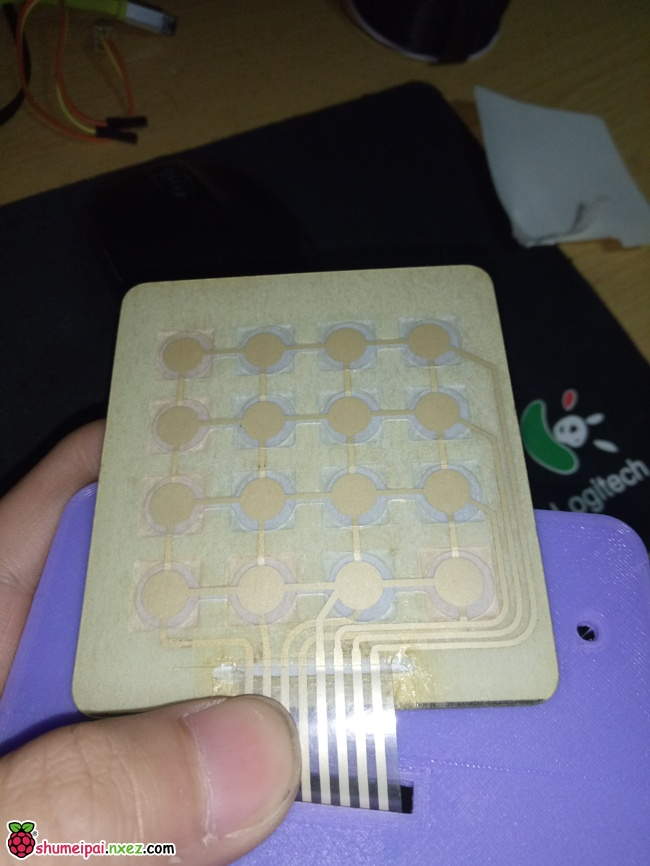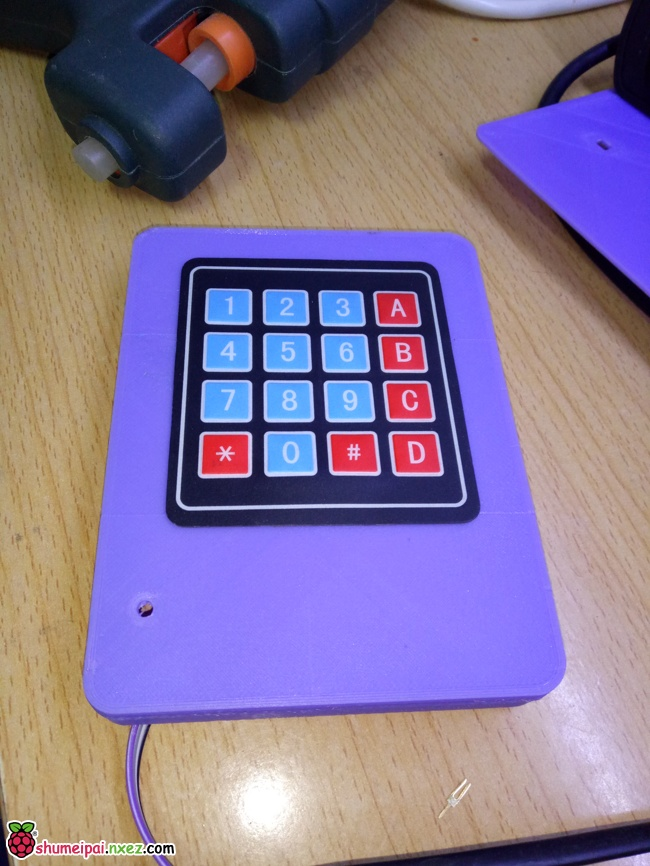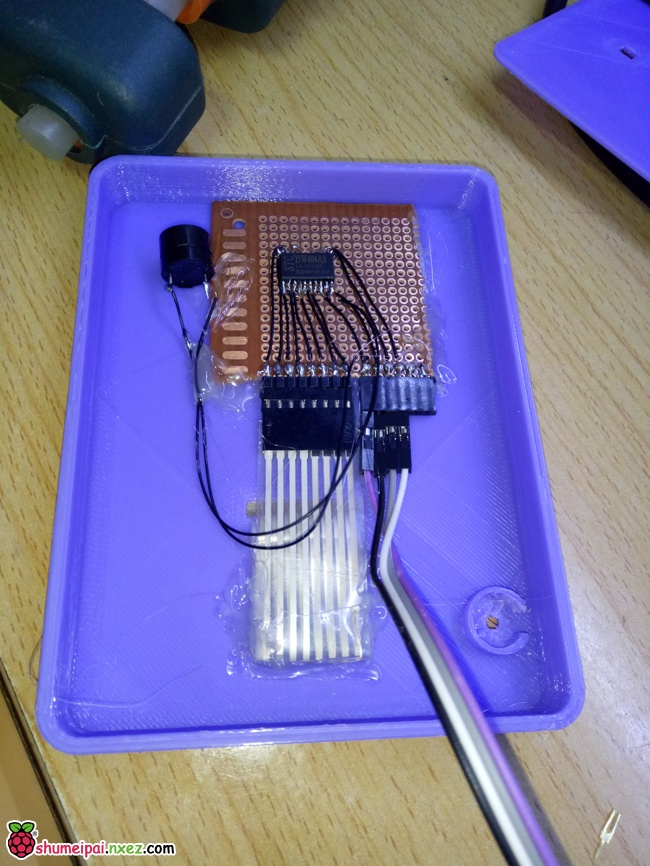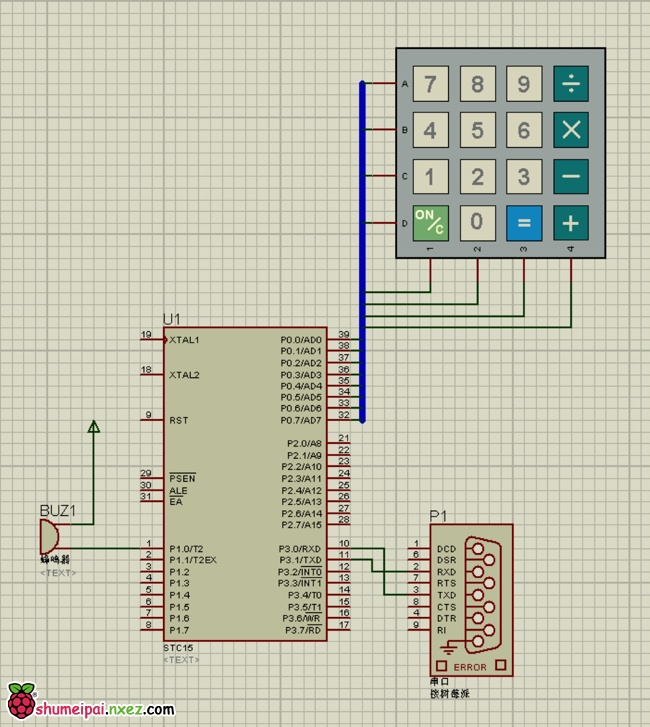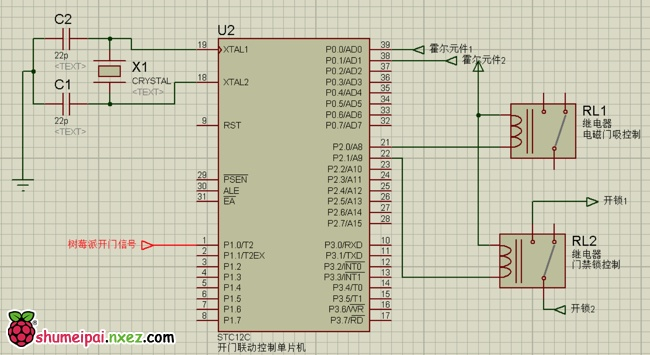(bilibili 吐槽请移步 http://www.bilibili.com/video/av9021381/)

https://shumeipai.nxez.com/2017/01/31/raspberry-serial-programming-python-example.html
https://shumeipai.nxez.com/2015/03/26/raspberry-pi-serial-programming-sending-and-receiving.html#### 14 评论

1. NB，好像弄一套

2. 作者用单片机读取键盘数据和开锁，树莓派负责逻辑处理并作为上位机控制这些单片机。语音 数据记录全在pi上。

3. 时钟的投影也是DIY的吗？还是已经有的成品？

4. 方便留个邮箱咨询么？最近正好在做这方面，有很多疑惑

5. 你好，请问树莓派如何接收并处理电脑上myeclipse编写的程序的结果，并实现门控功能呢，这样是否可行？

6. 你好，我最近在做人脸识别开锁，现在人脸识别已实现，请问下树莓派通过摄像头识别后，树莓派与锁通过什么实现门控功能？谢谢您！

• 您好，请问您人脸识别开锁作的怎么样了？我最近也想尝试，谢谢

7. 请问供电系统怎么接的?

8. 很厉害 最近刚接触到 树莓派的io 值的学习